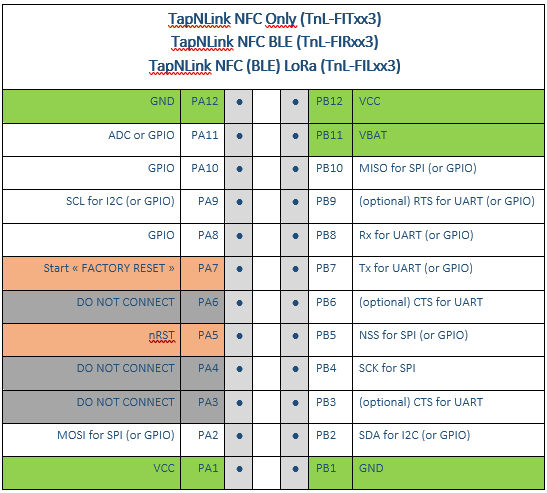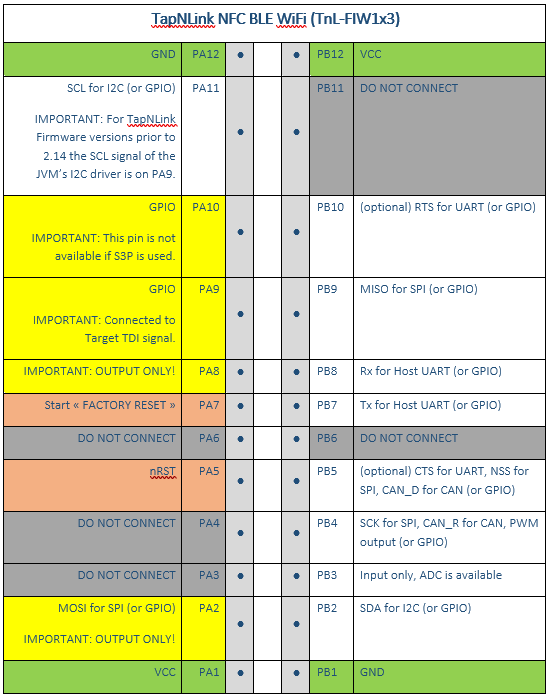# Java (native classes)

## IoTize specific

The IOT_JVM environment has several subclasses, detailed here, that give access to the TapNLink environment. They are implemented in the TapNLink firmware as native code, but they are not fully compliant with their official JAVA implementation (only a few methods are available).

• The TapNLinkVar object provides a simple synchronization between the variables of the IOTIZE STUDIO project and the IOT_JVM. Depending on the variable type (float, byte, int, array,…), various predefined subclasses exist (see \IoTize\IoTize Studio\bin\com\iotize\jvm).

• The Hal/Pin subclass provides a generic access to the GPIOs of the extension port. The pins addressability depends on the module. The end pins are always power pins, some pins are reserved, and some have different uses depending on the Tap. The “pin_id’ is an unsigned byte that specifies the reference of the pin on the extension ports:## “java/lang” classes

### Class java/lang/Integer

``````public Integer   valueOf(int i)
Returns an Integer instance representing the specified int value.

public Integer   valueOf(String s)
Returns an Integer object holding the value of the specified String.

public int       intValue()
Returns the value of this Integer as an int.
``````

### Class java/lang/Short

``````public Short     valueOf(short s)
Returns a Short instance representing the specified short value.

public Short     valueOf(String s)
Returns a Short object holding the value of the specified String.

public short     shortValue()
Returns the value of this Short as a short.
``````

### Class java/lang/Boolean

``````public Boolean   valueOf(boolean s)
Returns a Short instance representing the specified boolean value.

public Boolean   valueOf(String s)
Returns a Boolean object holding the value of the specified String.

public boolean   booleanValue()
Returns the value of this Boolean as a boolean.
``````

### Class java/lang/Byte

``````public Byte      valueOf(byte s)
Returns a Byte instance representing the specified byte value.

public Byte      valueOf(String s)
Returns a Byte object holding the value of the specified String.

public byte      byteValue()
Returns the value of this Byte as a byte.
``````

### Class java/lang/Character

``````public Character valueOf(char c)
Returns a Character instance representing the specified char value.

public char      charValue(char c)
Returns the value of this Character as a char.
``````

### Class java/lang/Long

``````public Long      valueOf(long s)
Returns a Long instance representing the specified long value.

public Long      valueOf(String s)
Returns a Long object holding the value of the specified String.

public long      longValue()
Returns the value of this Long as a long.

public double    doubleValue()
Returns the value of this Long as a double.
``````

### Class java/lang/Float

``````public Float     valueOf(float s)
Returns a Float instance representing the specified float value.

public Float     valueOf(String s)
Returns a Float object holding the value of the specified String.

public float     floatValue()
Returns the value of this Float as a float.

public int       floatToIntBits(float s)
Returns a representation of the specified floating-point value according to the IEEE 754 floating-point "single format" bit layout.

public float     intBitsToFloat(int s)
Returns the float value corresponding to a given bit representation.
``````

### Class java/lang/Double

``````public Double    valueOf(double s)
Returns a Double instance representing the specified double value.

public Double    valueOf(String s)
Returns a Double object holding the value of the specified String.

public double    doubleValue()
Returns the value of this Double as a double.

public double    longBitsToDouble(long s)
Returns the double value corresponding to a given bit representation.

public Long      doubleToLongBits(double s)
Returns a representation of the specified floating-point value according to the IEEE 754 floating-point "double format" bit layout.
``````

### Class java/lang/String

``````public String()
Initializes a newly created String object so that it represents an empty character sequence.

public String(byte[] bytes)
Constructs a new String by decoding the specified array of bytes using the platform's default charset.

public String(String original)
Initializes a newly created String object so that it represents the same sequence of characters as the argument; in other words, the newly created string is a copy of the argument string.

public String   format(String format, Object... args)
Returns a formatted string using the specified format string and arguments.

public int        length()
Returns the length of this string.

public char       charAt(int index)
Returns the char value at the specified index. An index ranges from 0 to length() - 1. The first char value of the sequence is at index 0, the next at index 1, and so on, as for array indexing.

public byte[]     getBytes()
Encodes this String into a sequence of bytes using the utf8 charset, storing the result into a new byte array.
Returns the resultant byte array

public char[]     toCharArray()
Returns this string to a new character array.

public boolean    equals(Object s)
Compares this string to the specified object.

public String     substring(int beginIndex)
Returns a new string that is a substring of this string.

public String     substring(int beginIndex, int endIndex)
Returns a new string that is a substring of this string.

public int        compareTo(String s)
Compares two strings lexicographically.

public String     hashCode()
Returns a hash code for this string.
``````

### Class java/lang/StringBuilder

``````public StringBuilder()
Constructs a string builder with no characters in it and an initial capacity of 16 characters.

public StringBuilder append:(String str)
Appends the specified string to this character sequence.
Returns a reference to the resulting object

public StringBuilder append(char i)
Appends the string representation of the char argument to this sequence.

public StringBuilder append(int i)
Appends the string representation of the int argument to this sequence. The overall effect is exactly as if the argument were converted to a string by the method String.valueOf(int), and the characters of that string were then appended to this character sequence.

public StringBuilder append(short i)
Appends the string representation of the short argument to this sequence. The overall effect is exactly as if the argument were converted to a string by the method String.valueOf(int), and the characters of that string were then appended to this character sequence.

public StringBuilder append(double i)
Appends the string representation of the double argument to this sequence. The overall effect is exactly as if the argument were converted to a string by the method String.valueOf(int), and the characters of that string were then appended to this character sequence.

public StringBuilder append(float i)
Appends the string representation of the float argument to this sequence. The overall effect is exactly as if the argument were converted to a string by the method String.valueOf(int), and the characters of that string were then appended to this character sequence.

public StringBuilder append(long i)
Appends the string representation of the long argument to this sequence. The overall effect is exactly as if the argument were converted to a string by the method String.valueOf(int), and the characters of that string were then appended to this character sequence.

public StringBuilder append(byte i)
Appends the string representation of the byte argument to this sequence. The overall effect is exactly as if the argument were converted to a string by the method String.valueOf(int), and the characters of that string were then appended to this character sequence.

public String     toString()
Returns a string representing the data in this sequence.

public int        length()
Returns the length (character count).

public char       charAt (int pos)
Returns the char value in this sequence at the specified index. The first char value is at index 0, the next at index 1, and so on, as in array indexing.
The index argument must be greater than or equal to 0, and less than the length of this sequence.
``````

### Class java/lang/Math

``````public double sin(double val)
Returns the sine of val; the returned angle is in the range 0.0 through pi.

public double cos(double val)
Returns the cosine of val; the returned angle is in the range 0.0 through pi.

public double acos(double val)
Returns the arc cosine of val; the returned angle is in the range 0.0 through pi.

public double asin(double val)
Returns the arc sine of val; the returned angle is in the range -pi/2 through pi/2.

public double tan(double val)
Returns the trigonometric tangent of an angle.

public double atan(double val)
Returns the arc tangent of val; the returned angle is in the range -pi/2 through pi/2.

public double exp(double val)
Returns Euler's number e raised to the power of a double value.

public double log(double val)
Returns the natural logarithm (base e) of a double value.

public double log10(double val)
Returns the base 10 logarithm of a double value.

public double abs(double val)
Returns the absolute value of a double value.

public double pow(double a, double b)
Returns the value of the first argument raised to the power of the second argument.

public double sqrt(double val)
Returns the correctly rounded positive square root of a double value.

public double floor(double val)
Returns the largest (closest to positive infinity) double value that is less than or equal to the argument and is equal to a mathematical integer.
``````

For all others classes, please refer to this document.